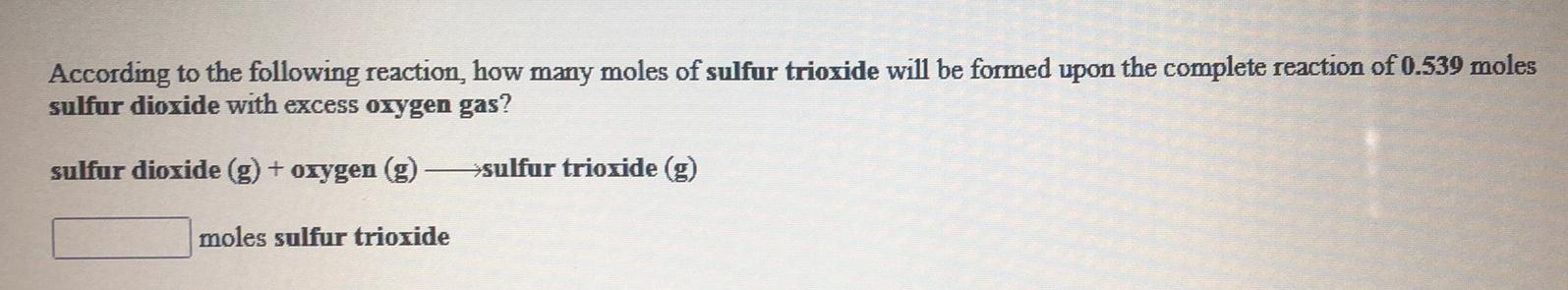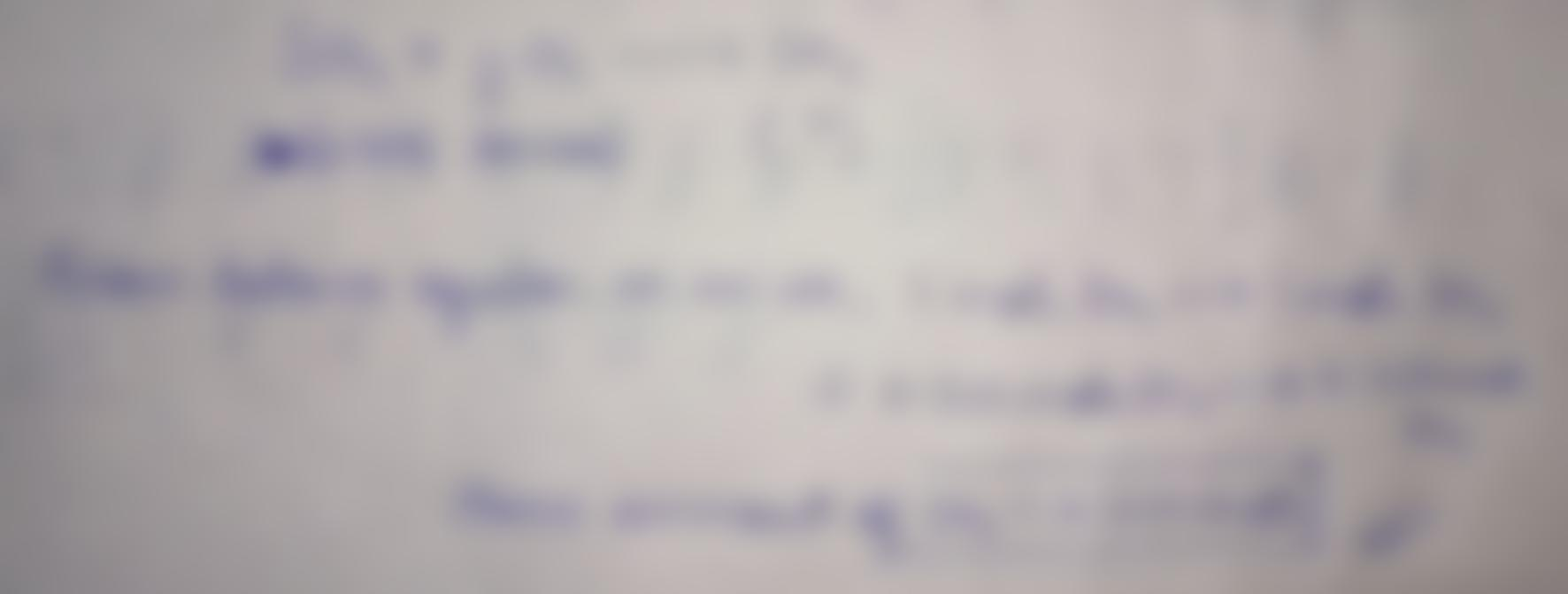Question:

According to the following reaction, how many moles of sulfur trioxide will be formed upon the complete reaction of 0.539 molesAccording to the following reaction, how many moles of sulfur trioxide will be formed upon the complete reaction of 0.539 moles sulfur dioxide with excess oxygen gas? sulfur dioxide (g) + oxygen (g) - sulfur trioxide (g) moles sulfur trioxide